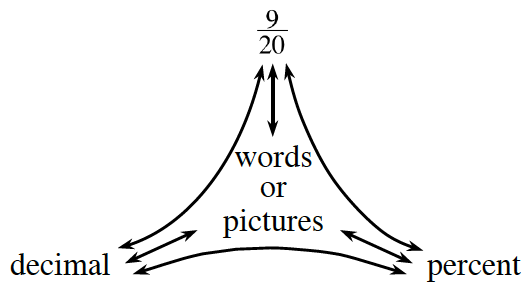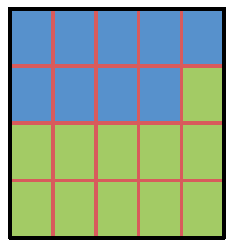Home > CC2 > Chapter 4 > Lesson 4.3.2 > Problem4-102

4-102.

Copy and complete the portions web below by including a picture, decimal, and percent representation of $\frac { 9 } { 20 }$. Homework Help ✎If the fraction given can be represented by the diagram below, how could you also write this fraction or diagram as a decimal and a percent?